# Divide

Divide the number 72 in the ratio 7: 2 and calculate the ratio of the numbers found in this order and write down as decimal.

a =  56
b =  16
p =  3.5

### Step-by-step explanation:

$p=a\mathrm{/}b=56\mathrm{/}16=\frac{7}{2}=3\frac{1}{2}=3.5$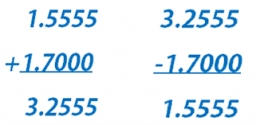Did you find an error or inaccuracy? Feel free to write us. Thank you!Tips to related online calculators
Need help to calculate sum, simplify or multiply fractions? Try our fraction calculator.
Check out our ratio calculator.

## Related math problems and questions:

• Divide 5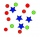Divide 288 in the following ratio 3 : 4 : 5
• Divide in ratioLine segment AB 12 cm long divide in a ratio of 5: 3. How long are the individual parts?
• David numberJana and David train the addition of the decimal numbers so that each of them will write a single number and these two numbers then add up. The last example was 11.11. David's number had the same number of digits before the decimal point, the Jane's numbe
• Divide 13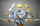Divide. Simplify your answer and write as an improper fraction or whole number. 14÷8/3
• Divide 11Divide the product of 4 and 5/8 by 1 1/2. Write your answer as a mixed number.
• Unknown numberIf I reduced the sum of the numbers 70 and the unknown number three times, I would get 100. what is the unknown number?
• Number ratio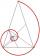Calculate two positive numbers that its ratio is 6:6 and difference was 0.
• Mushrooms from the forest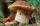Magda and Tereza go to pick mushrooms. Totally found 70 mushrooms. Magda found that between fungi found 5/9 bedel. Tereza discovered that she found among fungi are 2/17 champignons. How many mushrooms found, Magda?
• Unknown number4/5 of a number is 276. what is 2/3 of the same number?
• Two numbersThe difference between the two numbers is 74. If we divide a larger number by a smaller one, we get a quotient 7 and the rest of 2. Determine both numbers.
• Two-digit numberIn a two-digit number, the number of tens is three greater than the number of units. If we multiply the original number by a number written in the same digits but in reverse order, we get product 3 478. Find the original number.
• The number 72The number 72 increase by 25%. By how much % will you have to reduce the number you created to get the number 72 again?
• PeppersIn the box are yellow (a), green (b), and red (c) peppers. Their amount is in a ratio of 2:4:1. Most are yellow peppers and green the least. Calculate the number of peppers each type if the total number of peppers is 70.
• Division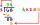Divide by the number 0.2 is the same as multiply by what number?
• Cylinder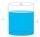The cylinder-shaped container has 80 liters of water. The water reaches 45 cm height. How many water liters will be in a container if the water level extends to a height of 72 cm? Write the result in liters, write down only as a whole or decimal number.
• Aspect ratioDetermine the ratio of the length to the width of a picture frame that is 12 3/4 inches long and 8 1/2 inches wide.
• Two numbersOne number is by 79 larger than the other. If we divide the larger number by the smaller one, we get the ratio 5 and the remainder 11. Determine both numbers.